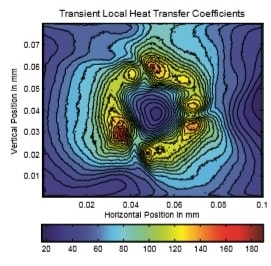# ME4611 Computing Group Project 2

Problem

A heated stainless steel foil is cooled by an axial fan as shown below. An Infrared Camera is placed directly above the foil and is used to measure the surface temperature in Kelvins. The experiment is carried out in steady state meaning that measurements are only recorded once the surface temperature of the foil does not change by 0.1 deg K over 15minutes. Temperatures recorded once steady state is reached are given in data.mat (available on SULIS. ). Data.mat consists of a number of arrays and matrices, the first is T which is a three dimensional matrix of size 256 x 320 x 61 where 256 represents the rows, 320 the columns and 61 the number of results i.e. the temporal measurement over 61 seconds. X is array of the x coordinates where max(X) gives the width of the foil, similarly Y is an array of the y coordinates where max(Y) gives the depth of the foil. (0,0) is the top left corner.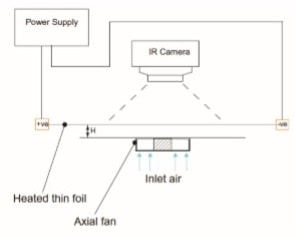In order to analyse this problem, energy balance can be applied to the experiment and is given as follows: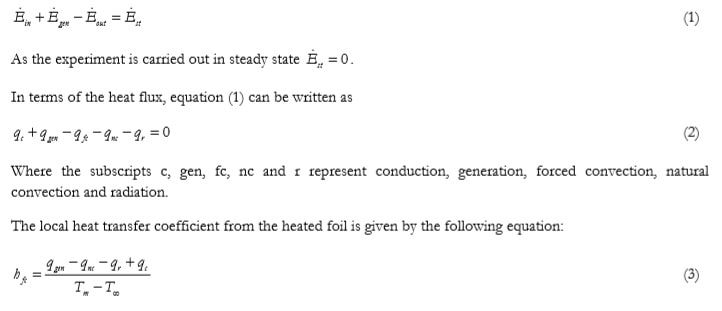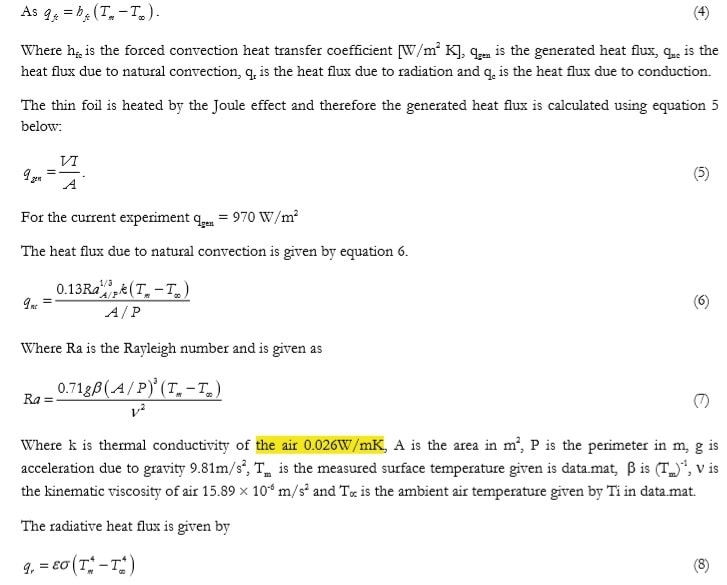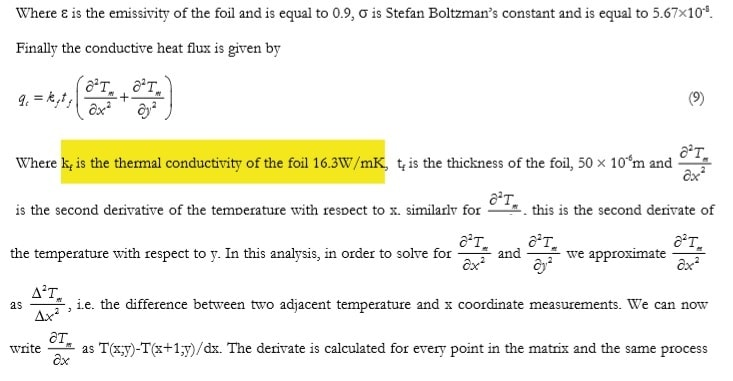applied again for the second derivate. This is known as numerical differentiation and can be written/coded in a number of different ways.